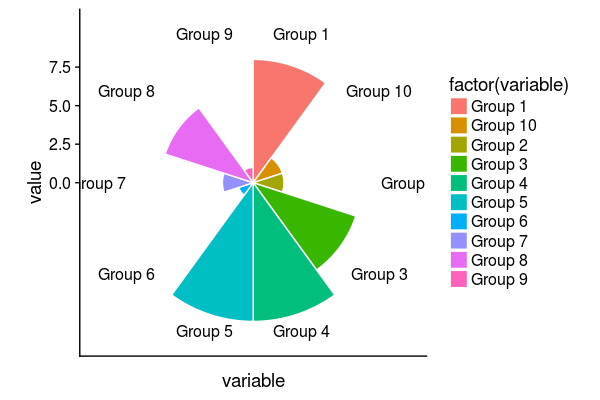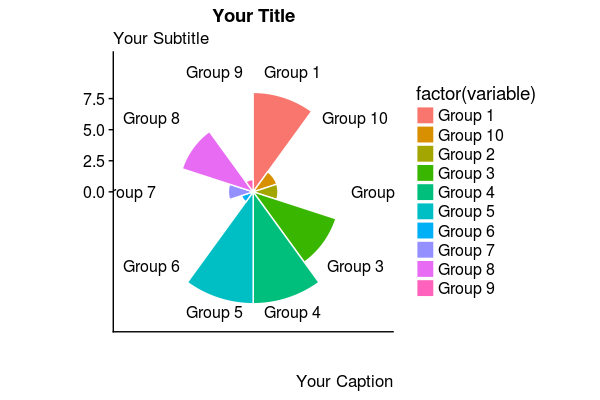# Creating Circumplex (Polar Bar) Charts in R with ggplot2While I was working on my last post about NFL salary cap allocation, I struggled with how best to present the information. I knew I wanted the focus to be on outliers, but was finding a standard pie chart to be dull, and bar chart to be hard to compare. Fortunately, I stumbled upon the circumplex chart and realized this was exactly what I was looking for.

I found a number of good resources to help me on my way, most notably this post by Learning R, but ultimately ended up heavily customizing the charts until they felt right. Let’s take a look at what it takes to build and customize one of these charts!

``````# Import tidyverse to have access to dplyr and ggplot2
library(tidyverse)

# Create a simple dummy data frame with columns "variable" and "value"
data <- data.frame(
variable = c(
"Group 1",
"Group 2",
"Group 3",
"Group 4",
"Group 5",
"Group 6",
"Group 7",
"Group 8",
"Group 9",
"Group 10"
),
value = sample(10, replace = TRUE))
``````

#### Create a Bar Plot

``````# Create a simple column chart using the data frame, colored by variable
plot <- ggplot(data,
aes(
x = variable,
y = value,
fill = factor(variable)
)) +
geom_col(width = 1, color = "white")

# View the plot
plot
``````#### Convert to Polar Plot

``````# Convert the coordinate system to polar
plot <- plot + coord_polar()

# View the plot
plot
````````````# Add descriptive text to the plot
plot <- plot + labs(
x = "",
y = "",
)

# View the plot
plot
``````#### Clean and Theme the Plot

``````# Clean up and theme the plot
plot <- plot +
# Assign the ggplot2 minimal theme
theme_minimal() +

# Remove legend, axes, text, and tick marks
theme(
legend.position = "none",
axis.title.x = element_blank(),
axis.title.y = element_blank(),
axis.ticks = element_blank(),
axis.text.y = element_blank(),
axis.text.x = element_text(face = "bold"),
plot.title = element_text(size = 24, face = "bold"),
plot.subtitle = element_text(size = 12)
)

# View the plot
plot
``````#### Save and Resize the Plot

``````ggsave("YOUR_FILENAME.png", width = 8, height = 6, unit = "in")
``````